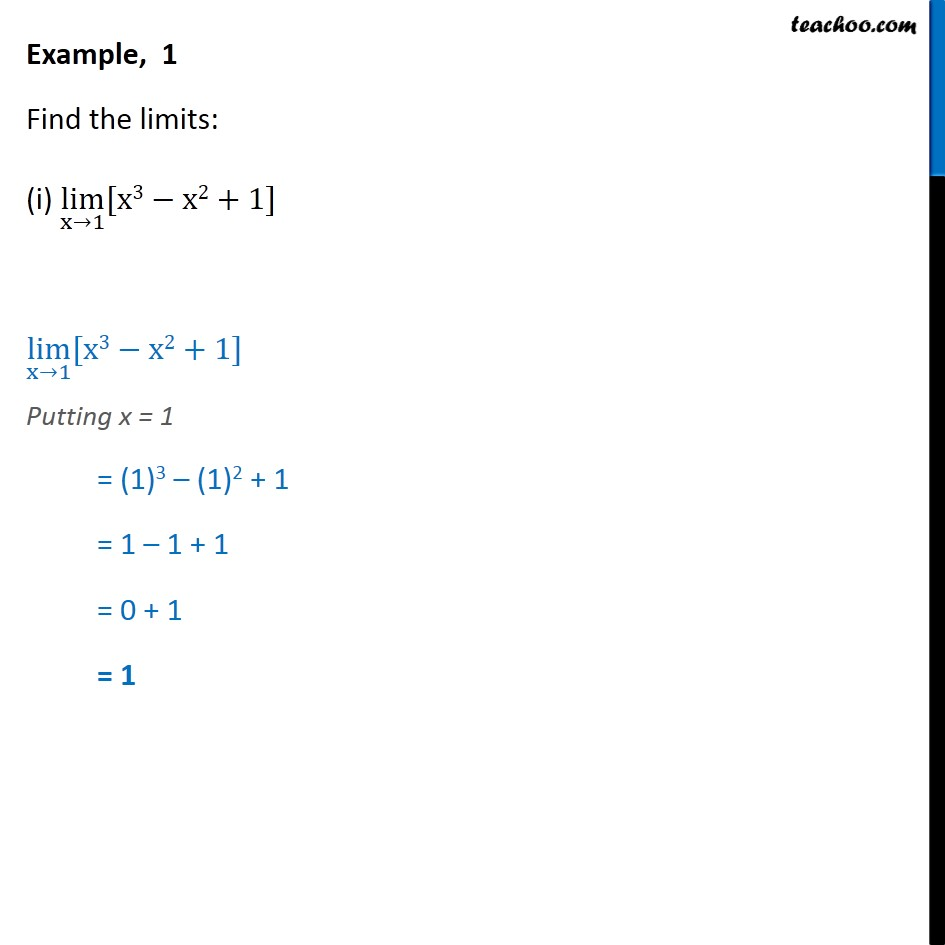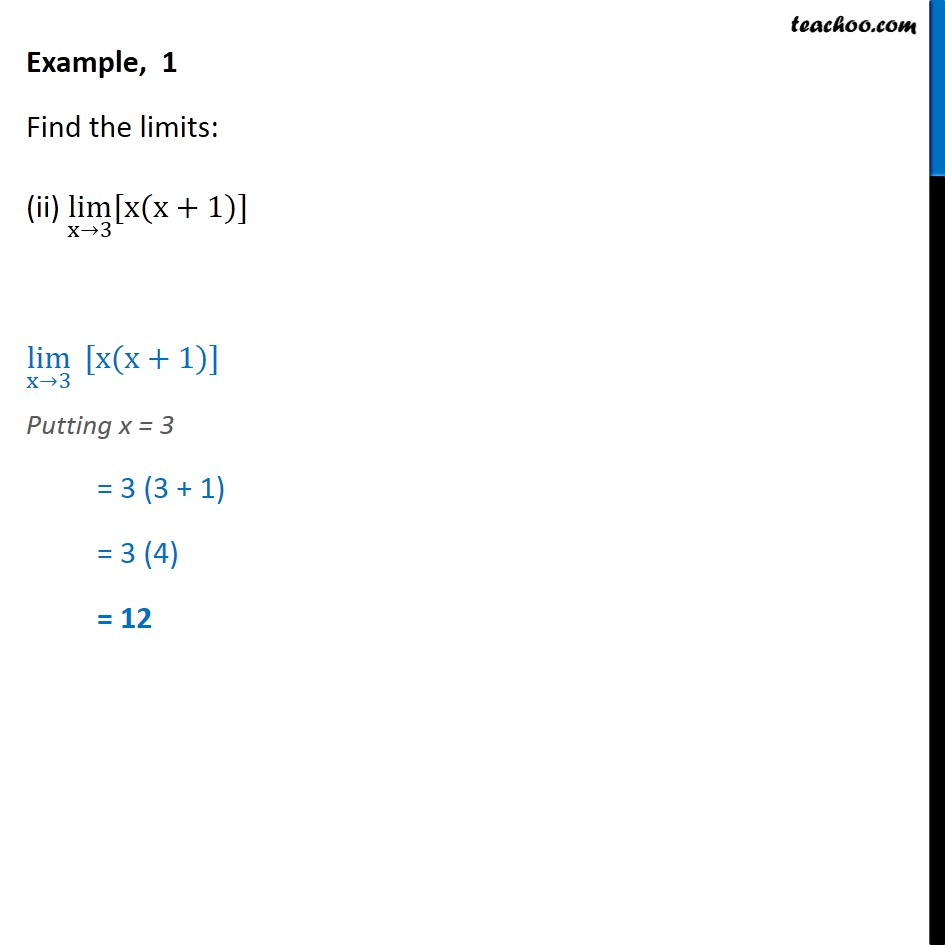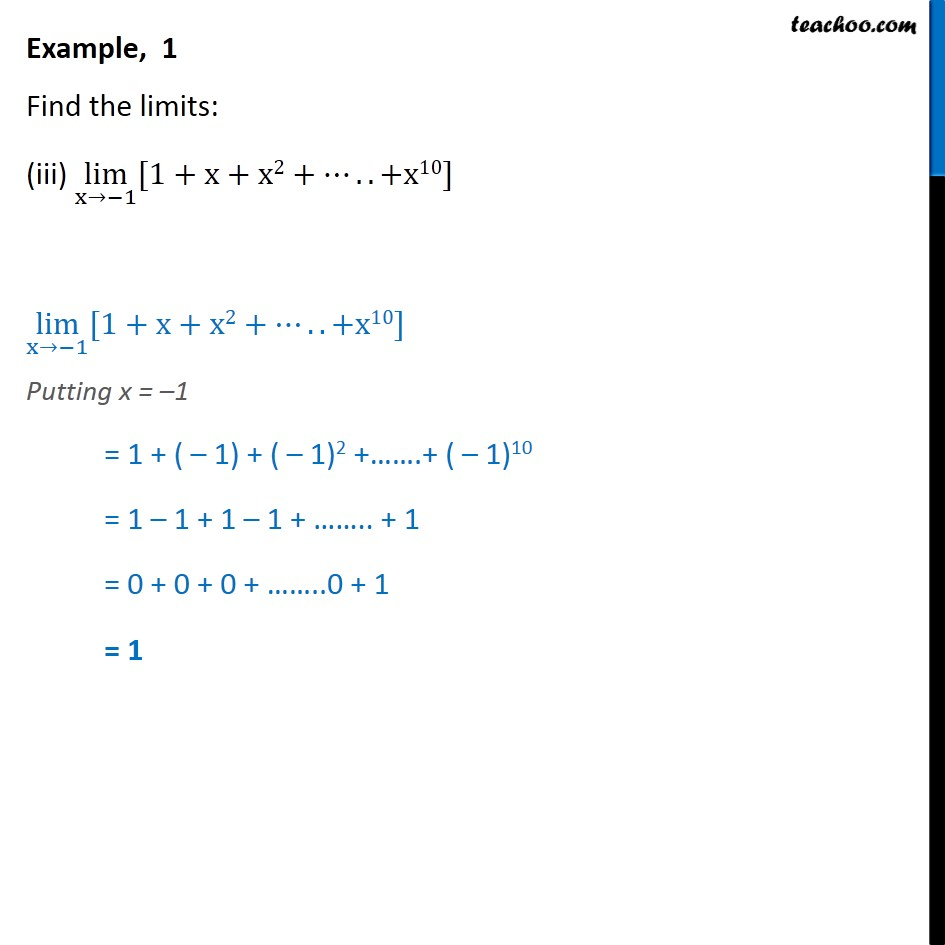1. Chapter 13 Class 11 Limits and Derivatives
2. Serial order wise
3. Examples

Transcript

Example, 1 Find the limits: (i) lim x 1 [x3 x2+1] lim x 1 [x3 x2+1] Putting x = 1 = (1)3 (1)2 + 1 = 1 1 + 1 = 0 + 1 = 1 Example, 1 Find the limits: (ii) lim x 3 [x(x+1)] lim x 3 [x(x+1)] Putting x = 3 = 3 (3 + 1) = 3 (4) = 12 Example, 1 Find the limits: (iii) lim x 1 [1+x+x2+ ..+x10] lim x 1 [1+x+x2+ ..+x10] Putting x = 1 = 1 + ( 1) + ( 1)2 + .+ ( 1)10 = 1 1 + 1 1 + .. + 1 = 0 + 0 + 0 + ..0 + 1 = 1

Examples